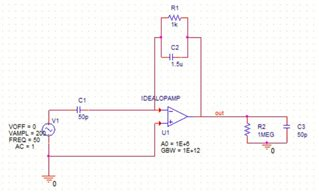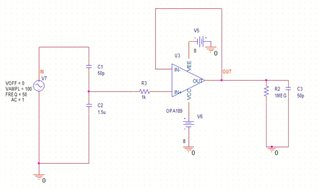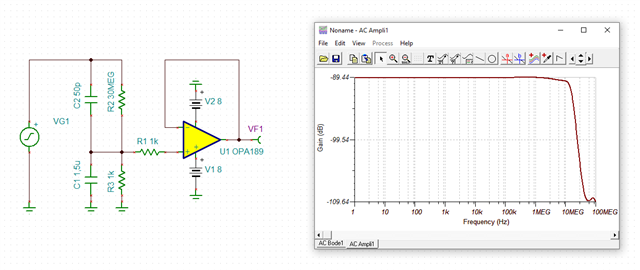This thread has been locked.

If you have a related question, please click the "Ask a related question" button in the top right corner. The newly created question will be automatically linked to this question.

# capacitive voltage divider interaction with operational amplifiers

Hi, I am working on developing a capacitive divider which would attenuate an AC voltage signal to a defined ratio. the circuit needs to have frequency bandwidth with constant attenuation in the range of 50Hz to 10kHz. It would be really helpful if someone can suggest me whether this would be possible to achieve from the circuit in the picture

thank you in advance.• Hi Imanka,

capacitive voltage dividers have the disadvantage of looking like a short circuit at high frequencies. Example given, the 50pF cap shows an impedance of 3R at 1GHz. So when an ESD is hitting the input of your circuit there would not be any current limitation and the OPAmp is in risk to become totally destroyed.

It would be better to place the capacitive input voltage divider at the +input of an non-inverting amplifier instead of placing it inside the feedback loop of an inverting amplifier.

Kai

• Thank you Kai, i have tried out another circuit as you have mentioned with the non-inverting configuration which is as shown in the figure. But this its frequency response changes with resistive load that is unavoidable. could you please suggest me some references which would help in solving this issue.• Hi Imanka,

you need a current path for the input bias current of OPAmp. Then, you should use a frequency compensated voltage divider like it can be seen in AC voltmeters or scope input voltage dividers:imanka_opa189_1.TSC

Kai

• Thank you Kai,

but my problem is that i cannot use a parallel resistor with the 50pF capacitor. i need to block dc voltages from the input source. is there a solution on this?

• Hi Imanka,

keep in mind that there's already the unavoidable isolation resistance of the 50pF cap in parallel to its capacitance.

You could either increase the involved resistances (R2, R3) by a certain factor or go for an isolation amplifier designhttps://www.ti.com/isolation/isolated-amplifiers/products.html

Kai

• thank you very much kai.

i will look into the possibility of using isolation amplifier for my circuit.

• Good luckKai